# Dynamic Analysis

Solution
• Click the banner below to learn how to apply Dynamic Analysis.

## 1. What is Dynamic Analysis?

One of the most crucial structural analyzes for bridge design is dynamic analysis. Static analysis is a state that does not changes over time because it ignores the effect of time and analyzes the state of equilibrium of forces in an object or system. Therefore, for static analysis problems, the shear force, moment, and deformation inside the member are determined by the size of a given load. Dynamic analysis can be said to be an analysis of the behavior of objects or systems, including changes over time (considering time or frequency).

Representatively, dynamic analysis applied to the design of bridges include dynamic moving load analysis of railway bridges and seismic design.

This page will explain the working principle and theoretical background of the functions used when performing dynamic analysis using a structural analysis program.

•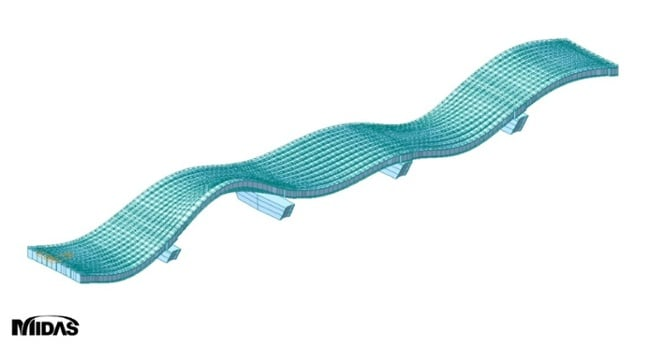Fig. Dynamic analysis

## 2. Dynamic Analysis for Seismic Design

In seismic design, seismic behavior analysis methods are divided into static analysis and dynamic analysis methods. Dynamic analysis methods include Response Spectrum Analysis and Time History Analysis specified by the Uniform Building Code (UBC). The most generalized method is the Response Spectrum analysis method. In order to use the response spectrum analysis, it is necessary to understand the design response spectrum, eigenvalue analysis and mode superposition method to calculate dynamic characteristics such as natural periods and mode shapes of structure.

•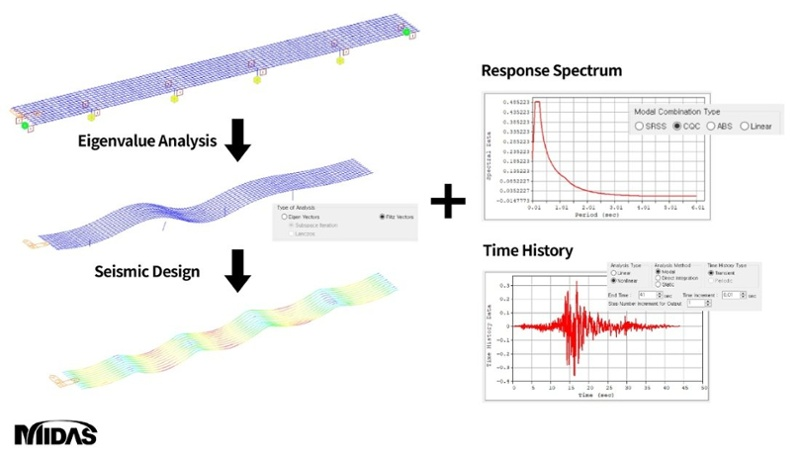Fig. Seismic design process

### A. Response Spectrum Analysis

Response Spectrum Analysis can be divided into single-mode spectrum analysis method and multi-mode spectrum analysis method depending on the analysis method. For complex structures such as bridges, multi-mode spectrum analysis method is used because several vibration modes affect the structure in a complex manner, and the response spectrum analysis in bridge analysis generally means a multi-mode spectrum analysis.

##### Single-Mode Spectrum Analysis

Also called Equivalent Static Analysis, it is a method applied to a structure with a regular shape where the stiffness and mass of the structure do not change significantly.

The effects of earthquakes are converted into Equivalent Static Forces using the fundamental period structures. In addition, by performing elastic analysis, member forces and displacements are obtained, and the seismic response of the entire structure is calculated.

### B. Design Response Spectrum

The Response Spectrum analysis assumes a Multi-Degree of Freedom (MDOF) as a complex Single-Degree of Freedom (SDOF), and sets the maximum response (displacement, velocity, acceleration) of the system as a function of the natural vibration period(Tm). The deformation, velocity, and acceleration, which are the maximum response values of the system, are expressed as a function of Tm, and are called deformation response spectrum, relative velocity response spectrum, and acceleration response spectrum, respectively.

##### Multi-Degree of Freedom

A typical structure is infinite number of degrees of freedom system formed by infinite material particles. The mass distributed in these systems can be calculated by assuming that such system is concentrated at several points. Such vibration systems are called MDOF structures.

For example, in the case of a rigid body motion, it has 3 degrees of freedom for translation motion and 3 degrees of freedom for rotational motion, resulting in a total of 6 degrees of freedom.

The design response spectrum used for seismic design of bridges is generally the design spectra defined in the design standards as shown in the figure below.

•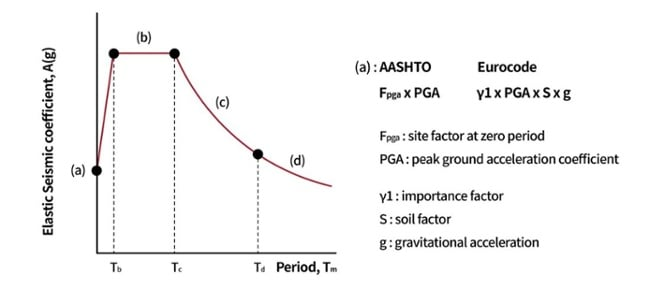Fig. Design response spectrum

AASHTO and Eurocode provide equations for calculating for Tb, Tc, Td, and its corresponding acceleration values, and the site factor (soil factor), importance factor, damping correction factor, spectral acceleration coefficient, and the peak ground acceleration coefficient are used as variables. However, careful consideration must be taken when using these specifications because the y-axis of the AASHTO spectrum is the acceleration (A) divided by the gravitational acceleration (g), and the Eurocode is the acceleration multiplied by the gravitational acceleration.

For seismic design, we obtain the acceleration values corresponding to the natural period of the structure obtained through the eigenvalue analysis from this spectrum. This value can be obtained from the maximum deformation of the structure and can be created through the same process as described below.

#### B-1. Equivalent Static Force

When the equation of motion is calculated through dynamic analysis, the member forces and stresses required for structural design can be determined through static analysis for each natural period of the structure. For this static analysis, the concept of equivalent static force is necessary.

Suppose that deformation u(t) occurs when an external force F(t) that changes over time acts on a structure with lateral stiffness (k) as shown in the figure below. By recording the deformations at every instant, the deformation response history can be recorded, and this process becomes the dynamic analysis.

•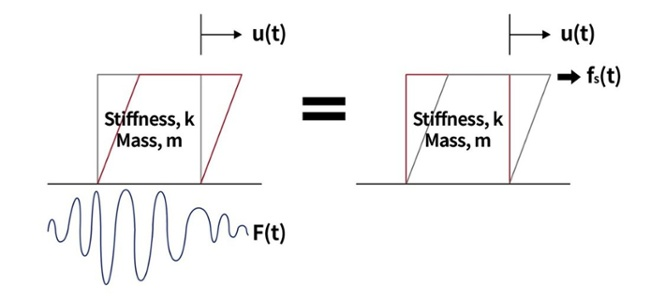Fig. Schematic of equivalent static force

F(t) can be defined as an external force that generates a deformation u(t) (obtained through dynamic analysis) in a structure with stiffness ‘k’. In other words, it is possible to define a static load capable of generating static deformation of the same size as the dynamic deformation u(t) in the structure at any given time (t), and this load is defined as the equivalent static load fs(t).

The ‘k’ in the equivalent static load equation can be expressed as a function of natural frequency, as shown below:

•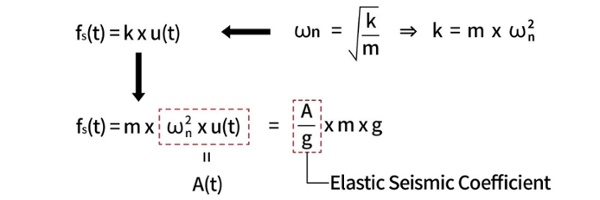Fig. A function of natural frequency

From this equation, the concept of pseudo-acceleration, A(t) can be obtained and expressed as a function of deformation and natural frequency. Note that the pseudo-acceleration is a value obtained from deformation, unlike the general concept of acceleration that represents a change in velocity. Furthermore, it can also be seen that the elastic seismic coefficient term is derived by modifying this equation.

##### Natural Frequency

When an external force is applied to a single-degree of freedom (SDOF) system without damping effect, it performs a simple harmonic motion in the form of a sine wave. The time it takes for a system to freely vibrate one cycle is called the natural period of vibration, and the unit is in seconds (sec). The natural frequency (ωn = 2π / T) with unit in rad/sec, has an inverse relationship with the natural vibration period Tm (natural frequency decreases as the natural period increase). If the undamped vibration system is assumed, it can be expressed as (ωn = √(k/m)), so the higher the stiffness of the structure, the faster the frequency, and inversely, the higher the mass, the lower the frequency.

#### B-2. Pseudo-Acceleration Response

The pseudo-acceleration response spectrum is the plot of the pseudo-acceleration as a function of the natural period of the structure. Here, the pseudo-acceleration can be determined from the equation for calculating A(t) and the peak deformation of the deformation response spectrum

The equation for calculating the pseudo-acceleration was derived from the equivalent static load, and the displacement response spectrum can be determined through the following process:

•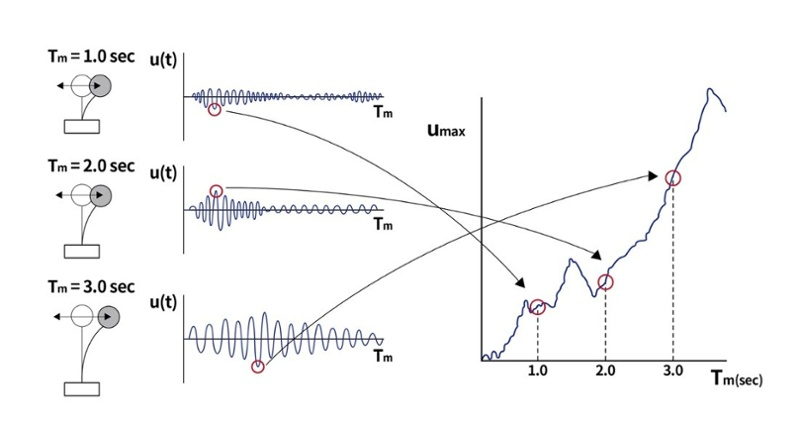Fig. Procedure to determine the deformation response spectrum

Assume the deformation response of three SDOF systems with constant damping ratio and different natural periods as shown in the figure above. The deformation response spectrum can be represented as a function of the natural vibration period and the maximum deformation of each ground motion system. Furthermore, it has physical properties that show that the longer the period, the greater the maximum deformation value.

It can also be seen from the equation that the pseudo-acceleration can be obtained by multiplying the maximum deformation by the square of the natural vibration period, and the deformation response spectrum can be converted to a pseudo-acceleration response spectrum.

•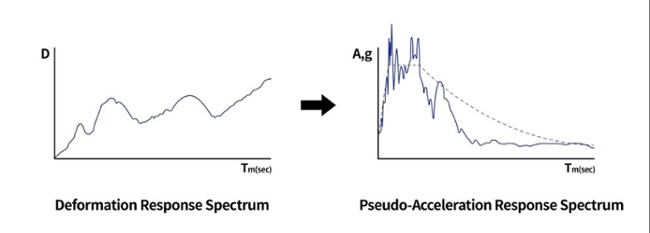Fig. Pseudo-acceleration response

From above figure, it can be seen that the shape of the pseudo-acceleration response spectrum is similar to that of the design response spectrum. Furthermore, it can also be seen that the response spectrum is related to the maximum deformation of the structure.

#### B-3. Combined Spectrum

The relationship between the maximum displacement and natural vibration periods obtained through the SDOF spectrum analysis can be expressed as follows. ‘A’, ’V’ and ‘D’ are the pseudo-acceleration, pseudo-velocity, and peak value of deformation, respectively.

A = ωn V = (ωn^2)D

As can be seen from the above equation, if the deformation response spectrum is known, the pseudo-velocity spectrum and the pseudo-acceleration spectrum can be obtained through algebraic operation. These three spectra only represent the structure’s response differently, but each provides physically meaningful values (‘A’, ‘V’, D are related to the maximum values of the equivalent static load and the base shear force, the maximum deformation energy stored in the structure during an earthquake, and the maximum displacement of the system, respectively). Furthermore, to make the design spectrum easy to calculate, a combination spectrum was developed by ‘Veletsos’ and ‘Newmark’.

The combination spectrum is a combination of three response spectrums on a four-way logarithmic paper.

•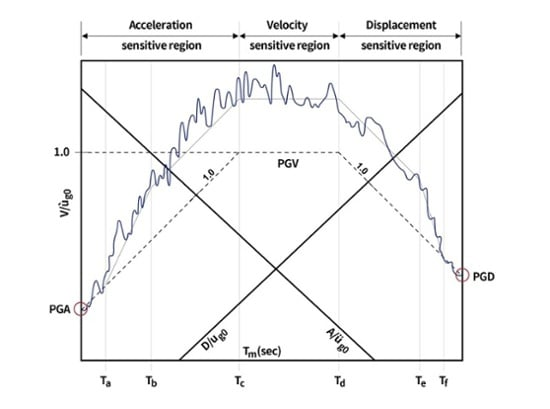Fig. Combined D-V-A response spectrum

We should pay attention to the information available from this graph. From this graph, it can be seen that there are sections where acceleration, velocity, and displacement are almost constant. The acceleration sensitive region is the region where the response of the structure is most directly related to the ground acceleration, the velocity-sensitive region is related to the ground velocity, and the displacement-sensitive region is most directly related to the ground displacement.

The two cases below explain how each section related to the response of a structure.

•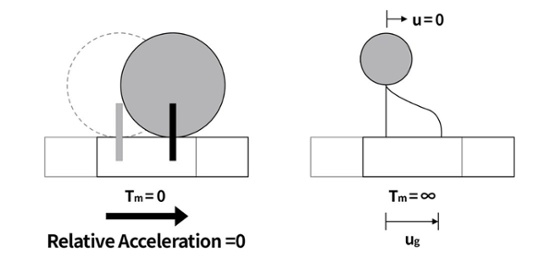Fig. Vibration models with extremely different natural vibration periods

The acceleration defined as the absolute value of a structure with a period of zero (the state where the ground and the structure are completely fixed and the relative displacement, relative velocity, and relative acceleration are all zero) converges to the peak ground acceleration. Conversely, in the case of infinitely periodic structures, the movement of the ground has no effect on the structure, so the relative deformation between the building and the ground becomes the same as the deformation of the ground itself. In other words, the displacement response of the structure does not diverge indefinitely, but converges to the peak ground displacement.

The location dividing each sensitive region can be defined, and the reference point that divides this region and location becomes the basis of the design spectrum. The design spectrum is a diagram that shows the functions of acceleration and period on an arithmetic scale, starting with PGA and continuing to the acceleration and velocity regions.

### C. Eigenvalue Analysis

Structures have natural vibration characteristics depending on their shape, material, and boundary conditions. Natural vibration characteristics refers to a free vibration state that does not receive any external forces. The analysis method to find these characteristics is called mode analysis, eigenvalue analysis, or free vibration analysis. Through the eigenvalue analysis, it is possible to know the natural frequency of the structure and the corresponding eigen-mode. The natural frequency represents the degree of how quickly it repeats per unit time, and the eigen-mode refers to a shape that can be freely deformed under a given constraint.

The natural vibration characteristics of the structure can be obtained from the undamped free vibration, which is a state that is not affected by external forces. Since it is an undamped free vibration, a dynamic equilibrium equation in which the damping matrix and external force are zero can be created. Furthermore, the natural frequency and eigen-mode can be calculated by assuming the displacement vector u(t) as the product of the displacement shape function and the time function. The MDOF system can be calculated in the same way as the SDOF system, except that the stiffness and mass are in matrix form.

The smallest natural frequency (longest natural vibration period) is referred to as the fundamental natural frequency of a structure, and is used as an important measurement of the dynamic characteristics of the structure. By knowing the natural frequency, corresponding mode shapes (free vibration mode, vibration mode or mode) can be obtained. The free vibration mode, which is the main problem of eigenvalues, represents only its shape as a combination of relative values, and assumes an absolute size as an arbitrary value. Therefore, since it is inconvenient to use the amplitude of the mode as an arbitrary value in numerical analysis, determining the amplitude of the mode shape according to a certain criterion is referred to as the normalization of the mode shape, and the mode obtained is called the normal mode.

The degree of freedom increases indefinitely as the analysis model of the structure becomes realistic. Since modes are created as much as the number of degrees of freedom the structure has, a situation arises in which a system of differential equations having as many unknowns as the number of degrees of freedom must be solved. Therefore, an appropriate degree of freedom should be selected and analyzed for efficient dynamic analysis, and one of the effective ways to reduce the degree of freedom is the mode superposition method using the eigenvector of the structure.

As mentioned above, accurately modeling the stiffness matrix in a structural analysis model that is similar to the actual behavior results in a greater degree of freedom. The mode superposition method does not consider all of these degrees of freedom in the dynamic analysis, but is a method of increasing the efficiency by including only the eigenvector or Ritz Vector, which has a major effect on the dynamic analysis. The figure below shows the basic concept of the mode superposition method, and shows that the deformed shape of a structure with multiple degrees of freedom can be expressed with only one representative mode.

•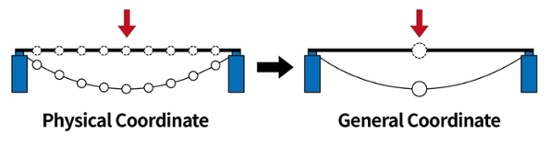Fig. Basic concept of the mode superposition method (selection of degree of freedom)

In general, the mode superposition method uses eigenvectors to solve equations of motion. In this case, since all of the matrix terms become diagonal matrices, each degree of freedom is transformed into an uncoupled ordinary differential equation.

##### Subspace iteration method

Subspace Iteration matrix calculation is used to perform eigenvalue analysis. This method is effectively used when performing eigenvalue analysis for a finite element system of a large scale (large matrix system)

##### Lancozos iteration Method

Tridiagonal Matrix is used to perform eigenvalue analysis. This method is effectively used when performing eigenvalue analysis for lower modes.

##### Ritz method

The Ritz method is used to obtained the natural frequency after replacing it with a SDOF structure by assuming the mode shape of the MDOF structure. As an extension of the Rayleigh-Ritz method, an improved Ritz vector can be obtained through an iterative calculation process using inertial forces. Even though the displacement of a structure can be approximated by some shape, it is assumed that the displacement is approximated by several Ritz vectors instead of one shape. By using the eigenvalues reflecting the characteristics of the dynamic loads, it is possible to obtain more accurate results than the general eigenvectors with relatively small number of modes.

### D. Modal Combination Type

Response spectrum analysis is performed using the natural vibration period and the elastic earthquake response coefficient of the structure obtained through the eigenvalue analysis. The member forces and displacements are obtained by combining the values obtained in the individual modes.

Since the maximum values for each mode do not occur at the same time, individual mode responses can be superimposed by ‘Square Root of the Sum of the Squares’ (SRSS) or ‘Compete Quadratic Combination’ (CQC) method to calculate the maximum values of member forces and displacement.

•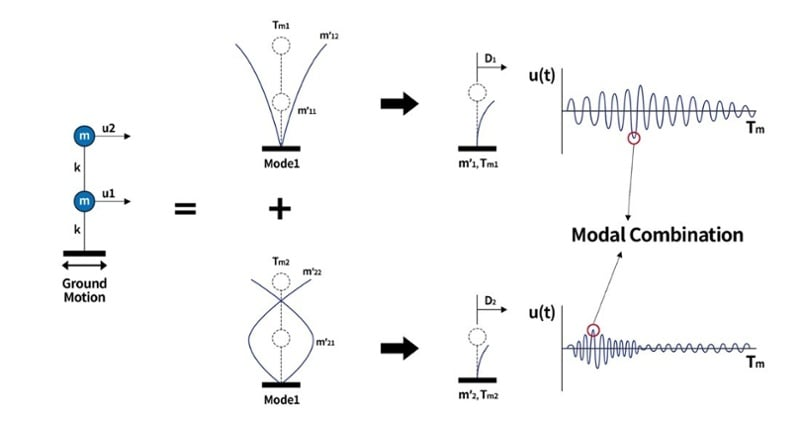Fig. Modal combination concept

In general, the SRSS method can be applied to all types of bridges, but it is known that large errors may occur in bridges where the spacing between modes is narrow due to the special geometry of the bridge. For the combined response of such bridges, there is the CQC method in which the contribution of each mode is weighted and combined.

##### SRSS (Square Root of the Sum of the Squares)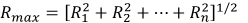The SRSS method, which is the most commonly used method, renders close approximations of design response for a structure exhibiting well-distributed natural frequencies. However, it tends to overestimate or underestimate the combination for a structural system with close natural frequencies, which can be found in a multi-span bridge with continuous short spans. Another drawback is that it loses signs in the process of combination.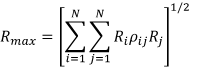The CQC method considers the probabilistic correlation between modes for a structural system with close natural frequencies, which can be found in a multi-span bridge with continuous short spans. By applying the correlation factor in combination using close natural frequency ratios, the overestimation or underestimation problem can be resolved. If the damping ratio is zero, it is the same as the results of SRSS.

##### ABS (Absolute Sum)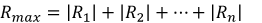The ABS method renders the largest responses among different combination methods. The signs are neglected by the use of absolute values. It tends to overestimate the response results. When a specific ratio such as the 100:30 rule is applied after combining the analysis results in each direction considering the directionality of earthquakes, the maximum response is obtained by summing the absolute results in three directions.

The disadvantage of mode-specific combination methods (SRSS, CQC, ABS) is that the effect of signs (positive, negative) between modes cannot be considered. This result affects the sign of the member force for the main member, causing the problem of being overestimated or underestimated in the combination of the member force with the forces that the direction of gravity. This can be said to be a fundamental problem of the response spectrum analysis modal combination method. An alternative must be considered when dynamic analysis using a structural analysis program.

For example, in the case of MIDAS civil, a method of calculating the combination result using the sign of the major mode that contributes the most to the behavior of the structure is proposed. For most structures, the mass participation rate or contribution of the 1st mode for each direction is the largest, so if the combination is performed in consideration of the sign of the 1st mode for each direction, errors in the combination result can be resolved to some extent. However, the effects of higher-order mode may be significant if the structure is highly irregular or torsional, making it difficult to distinguish the main mode. In this case, it is preferable to apply the sign for the mode with the greatest absolute value among the results for each mode.

## 3. Dynamic Analysis of Railway Bridge

It is necessary to secure structural stability and serviceability of railway bridges through dynamic analysis reflecting the continuous and periodic load characteristics of trains. The dynamic analysis of railway bridges is to examine the possibility of resonance occurrence by matching the natural frequency of the bridge with the frequency of the train’s effective strike interval.

•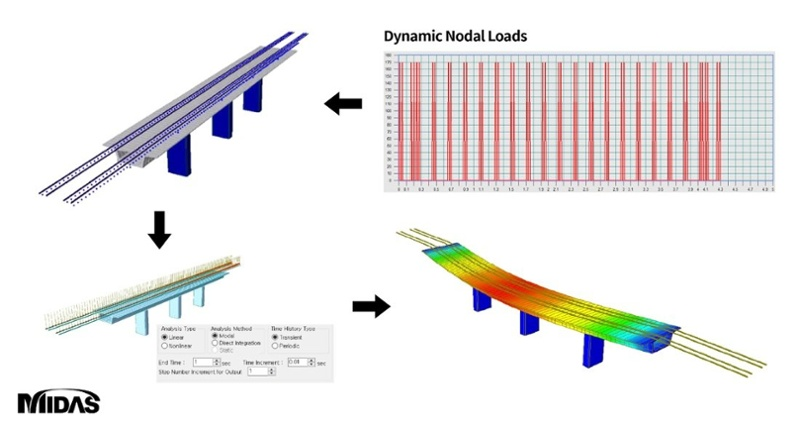Fig. Dynamic analysis of railway bridge

The critical speed that can cause resonance can be estimated in advanced by the product of the natural frequency of the 1st vertical mode obtained through the eigenvalue analysis and the effective strike distance of the train. Moreover, an interpretation of this speed should also be included in the analysis.

With the results of the dynamic analysis of trains, it is necessary to review the structural stability as well as the limitations on the maximum vertical deflection, maximum vertical acceleration, and maximum end-rotation angle.

### A. Time History Analysis

The Time History Analysis method is used to calculate the actual behavior (displacement, member forces, etc) of the structure at any given time using the dynamic characteristics of the structure and the external forces applied, and is used when the distinction between modes is not clear or nonlinear analysis is required.

Time history analysis methods include the ‘Mode Superposition Method’ and the ‘Direct Integration Method’. When the time history analysis is used for seismic analysis, it is an accurate analysis method that can find out the behavior of a structure when the change in the ground motion caused by an earthquake over time is known, but it has a disadvantage that it is difficult to accurately predict the expected ground motion. In seismic analysis, as mentioned above, the response spectrum analysis method more commonly used, and for railway bridge dynamic analysis, which has periodic loads, the time history analysis should be performed.

In the time history analysis, the time variable is very important. The analysis time should be enough for the train to pass completely from the start to the end of the bridge. Since the time interval is a variable that significantly affects the accuracy of the analysis results that are closely related to the period of the higher-order mode and the period of the load, it is necessary to check the values suggested by the design criteria or the structural analysis program.

##### Direct Integration Method

A method of obtaining the structure’s behavior over an entire time interval by repeating the process of obtaining the structure’s behavior at the next point in time when the behavior at one point in time is obtained.

##### Mode Superposition Method

A method of obtaining the theoretically accurate response time history analysis by dividing the structure’s behavior into the behavior of each mode and superimposing the responses in all modes.

The load function inputted in the analysis is calculated according to the length of the element and the distance between the vehicle wheels, and is then entered to each node according to the arrival time.

Multiple loads should not be superimposed onto one element.

Although the train load can be defined using a structural analysis program, moving from i to i+1 is considered loading, and moving from i+1 to i+2 is considered unloading, as shown in the figure below, and can be written as a triangular pulse load.

•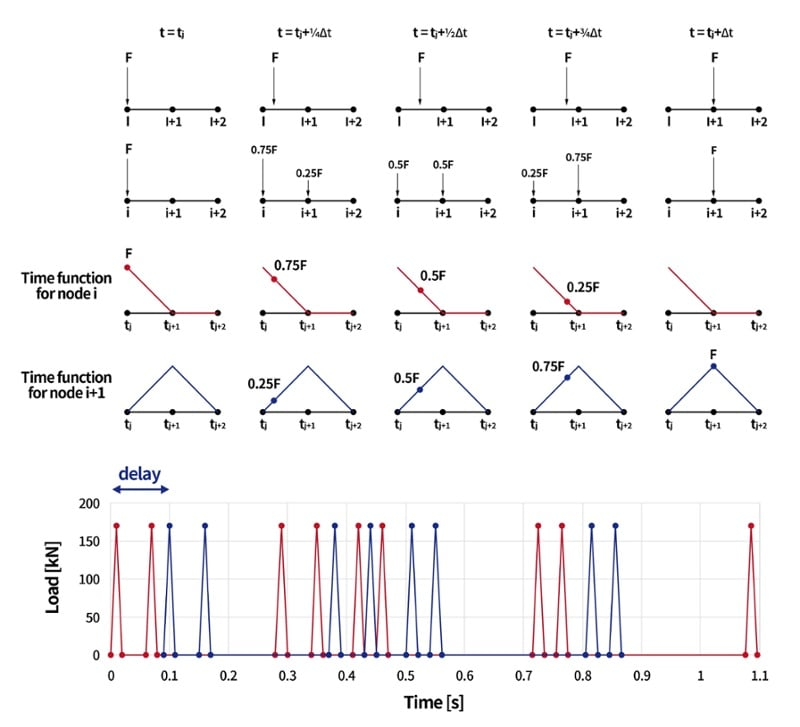Fig. How to transform a moving load to dynamic loads using time function